# AP Statistics : How to find p-values

## Example Questions

### Example Question #1 : How To Find P Values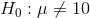and the sample mean is 12.

Select the answer so that both statements indicate a rejection of the null hypothesis at the 95% confidence level.

1) The Z-value for 12 is less than 1.96

and

2) The p-value for 12 is greater than .05

1) The Z-value for 12 is greater than 1.96

and

2) The p-value for 12 is less than .05

1) The Z-value for 12 is greater than 1.96

and

2) The p-value for 12 is greater than .05

1) The Z-value for 12 is less than 1.96

and

2) The p-value for 12 is less than .05

1) The Z-value for 12 is greater than 1.96

and

2) The p-value for 12 is less than .05

Explanation:

In order to reject the null hypothesis, the Z-value for the sample must be greater than (i.e. must lie outside of) the Z-value of the confidence level.

By definition, if the Z-value of the sample is greater than the Z-value of the confidence level, then the p-value of the sample must be less than the p-value for the confidence level.

### Example Question #2 : How To Find P Values

Under the null hypothesis, the distribution of a stock price is normal with meanand standard deviation. The actual stock price now is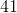. What is the probability that the stock price is this much or greater under the null hypothesis?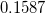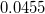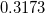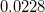Explanation:

This exercise consists of computing the p-value. The null distribution is normal, so we must compute the z-score with the actual data we have and use it to compute the p-value.

We have: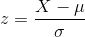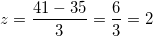Now we calculate the chance that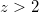, using the context from the problem.

Using a normal table, we get, which is the answer.

### Example Question #3 : How To Find P Values

We are testing the hypothesis that the average gas consumption per day in Billings, Montana is greater than 7 gallons per day; we want 95% confidence.

We sample 30 drivers. The average is 8.4, and the sample standard deviation is 4.29.

Our null hypothesis is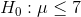1) What is the Z-value for a 1-tailed test at 95%?

2) What is the Z-value for our sample mean of 8.4?

3) What is the p-value for our sample mean of 8.4?

4) Do we reject the null hypothesis?

1) 1.64

2) 1.9

3) .028

4) Yes

1) 1.64

2) 1.95

3) .020

4) Yes

1) 1.64

2) 1.8

3) .036

4) Yes

1) 1.64

2) 1.55

3) .067

4) No

1) 1.64

2) 1.44

3) .083

4) No

1) 1.64

2) 1.8

3) .036

4) Yes

Explanation: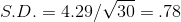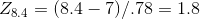From the Z-table: 1.8 corresponds to .9641; p= 1 - .9641 = .036

We reject the null hypothesis since 1.8 > 1.64 (and .036 is less than 95%).

In plain English, we are 95% sure that we will not get a sample mean of 8.4 when the true population mean is 7.